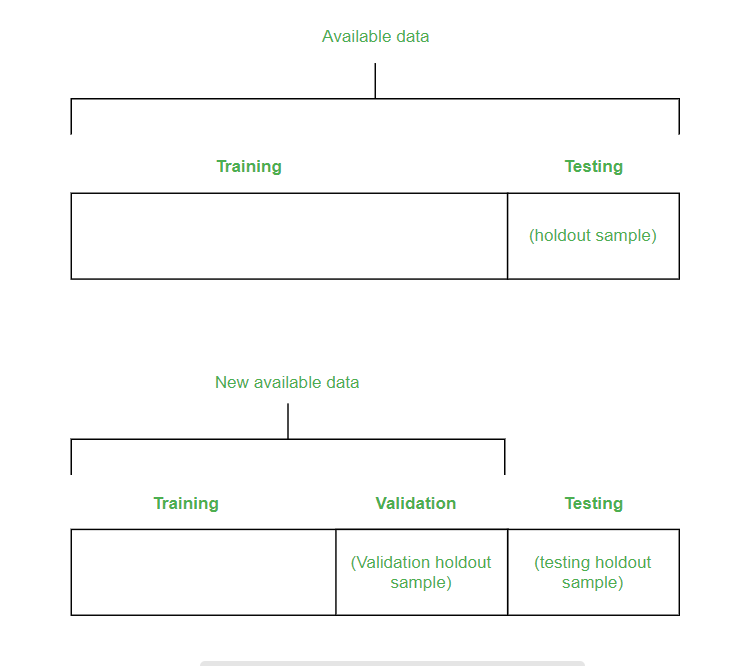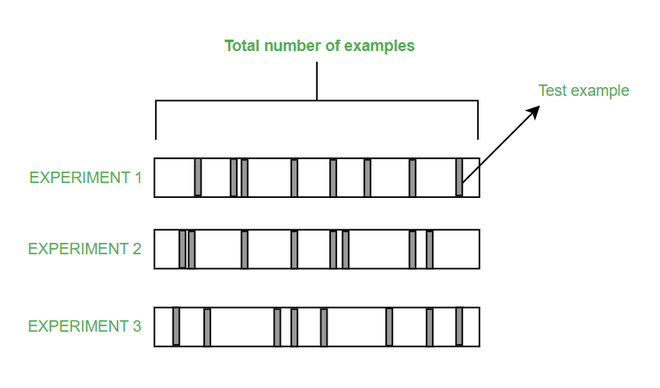# Techniques To Evaluate Accuracy of Classifier in Data Mining

Pre-requisites: Data Mining

Data Mining can be referred to as knowledge mining from data, knowledge extraction, data/pattern analysis, data archaeology, and data dredging.  In this article, we will see techniques to evaluate the accuracy of classifiers.

### HoldOut

In the holdout method, the largest dataset is randomly divided into three subsets:

• A training set is a subset of the dataset which are been used to build predictive models.
• The validation set is a subset of the dataset which is been used to assess the performance of the model built in the training phase. It provides a test platform for fine-tuning of the model’s parameters and selecting the best-performing model. It is not necessary for all modeling algorithms to need a validation set.
• Test sets or unseen examples are the subset of the dataset to assess the likely future performance of the model. If a model is fitting into the training set much better than it fits into the test set, then overfitting is probably the cause that occurred here.

Basically, two-thirds of the data are been allocated to the training set and the remaining one-third is been allocated to the test set.### Random Subsampling

• Random subsampling is a variation of the holdout method. The holdout method is been repeated K times.
• The holdout subsampling involves randomly splitting the data into a training set and a test set.
• On the training set the data is been trained and the mean square error (MSE) is been obtained from the predictions on the test set.
• As MSE is dependent on the split, this method is not recommended. So a new split can give you a new MSE.
• The overall accuracy is been calculated as E = 1/K \sum_{k}^{i=1} E_{i}### Cross-Validation

• K-fold cross-validation is been used when there is only a limited amount of data available, to achieve an unbiased estimation of the performance of the model.
• Here, we divide the data into K subsets of equal sizes.
• We build models K times, each time leaving out one of the subsets from the training, and use it as the test set.
• If K equals the sample size, then this is called a “Leave-One-Out”

### Bootstrapping

• Bootstrapping is one of the techniques which is used to make the estimations from the data by taking an average of the estimates from smaller data samples.
• The bootstrapping method involves the iterative resampling of a dataset with replacement.
• On resampling instead of only estimating the statistics once on complete data, we can do it many times.
• Repeating this multiple times helps to obtain a vector of estimates.
• Bootstrapping can compute variance, expected value, and other relevant statistics of these estimates.
Whether you're preparing for your first job interview or aiming to upskill in this ever-evolving tech landscape, GeeksforGeeks Courses are your key to success. We provide top-quality content at affordable prices, all geared towards accelerating your growth in a time-bound manner. Join the millions we've already empowered, and we're here to do the same for you. Don't miss out - check it out now!

Previous
Next